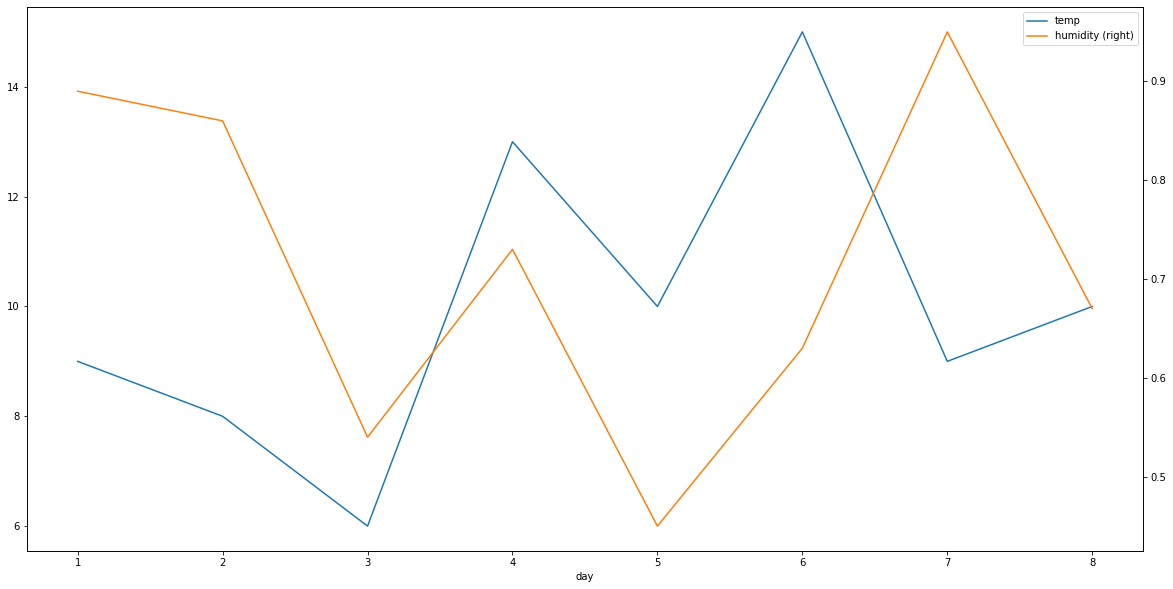To plot two variables on two sides of Y-axes, we can plot in two steps:

• plot first variable on the main y-axis - left one
• plot the second variable on the secondary y-axis - right one

## Steps to plot 2 variables

• Import matplotlib library
• Create DataFrame with correlated data
• Create the figure and axes object - `fig, ax = plt.subplots()`
• Plot the first variable on x and left y axes
• Plot the second variable on x and secondary y axes

## Data

day temp humidity
0 1 9 0.89
1 2 8 0.86
2 3 6 0.54
3 4 13 0.73
4 5 10 0.45

## Example

``````import pandas as pd
import matplotlib.pyplot as plt

df = pd.DataFrame(data={'day': [1, 2, 3, 4, 5, 6, 7, 8],
'temp': [9, 8, 6, 13, 10, 15, 9, 10],
'humidity': [0.89, 0.86, 0.54, 0.73, 0.45, 0.63, 0.95, 0.67]})

fig, ax = plt.subplots(figsize=(20,10))

df.plot(x = 'day', y = 'temp', ax = ax)
df.plot(x = 'day', y = 'humidity', ax = ax, secondary_y = True)
``````

## Output